# NCERT Solutions For Class 11 Chemistry chapter 4-Chemical Bonding And Molecular Structure

NCERT Solutions for class-11 Chemistry Chapter 4 Chemical Bonding and Molecular Structure is prepared by our senior and renown teachers of Physics Wallah primary focus while solving these questions of class-11 in NCERT textbook, also do read theory of this Chapter 4 Chemical Bonding and Molecular Structure while going before solving the NCERT questions. Our Physics Wallah team Prepared Other Subjects NCERT Solutions for class 11.

### Answer the following Questions.

1. Explain the formation of a chemical bond.

Solution :

A chemical bond is defined as an attractive force that holds the constituents (atoms, ions etc.) together in a chemical species.

Various theories have been suggested for the formation of chemical bonds such as the electronic theory, valence shell electron pair repulsion theory, valence bond theory, and molecular orbital theory.

A chemical bond formation is attributed to the tendency of a system to attain stability. It was observed that the inertness of noble gases was because of their fully filled outermost orbitals. Hence, it was postulated that the elements having incomplete outermost shells are unstable (reactive). Atoms, therefore, combine with each other and complete their respective octets or duplets to attain the stable configuration of the nearest noble gases. This combination can occur either by sharing of electrons or by transferring one or more electrons from one atom to another. The chemical bond formed as a result of sharing of electrons between atoms is called a covalent bond. An ionic bond is formed as a result of the transference of electrons from one atom to another.

2. Write Lewis dot symbols for atoms of the following elements: Mg, Na, B, O, N, Br.

Solution :

Mg: There are two valence electrons in Mg atom. Hence, the Lewis dot symbol for Mg is: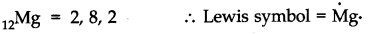Na: There is only one valence electron in an atom of sodium. Hence, the Lewis dot structure is: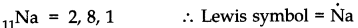B: There are 3 valence electrons in Boron atom. Hence, the Lewis dot structure is: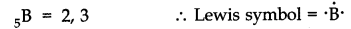O: There are six valence electrons in an atom of oxygen. Hence, the Lewis dot structure is: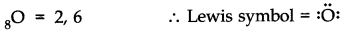N: There are five valence electrons in an atom of nitrogen. Hence, the Lewis dot structure is: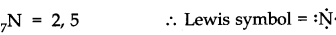Br: There are seven valence electrons in bromine. Hence, the Lewis dot structure is: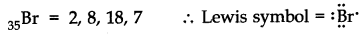3. Write Lewis symbols for the following atoms and ions:

S and S2–; Al and Al3+; H and H

Solution :

(i) S and S2–

The number of valence electrons in sulphur is 6.

The Lewis dot symbol of sulphur (S) is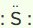.

The dinegative charge infers that there will be two electrons more in addition to the six valence electrons. Hence, the Lewis dot symbol of S2– is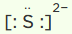.

(ii) Al and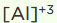The number of valence electrons in aluminium is 3.

The Lewis dot symbol of aluminium (Al) is.AI

The tripositive charge on a species infers that it has donated its three electrons. Hence, the Lewis dot symbol is.

(iii) H and H–

The number of valence electrons in hydrogen is 1.

The Lewis dot symbol of hydrogen (H) is H.

The uninegative charge infers that there will be one electron more in addition to the one valence electron. Hence, the Lewis dot symbol is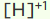.

4. Draw the Lewis structures for the following molecules and ions:

H2S, SiCl4, BeF2,CO32-, HCOOH

Solution :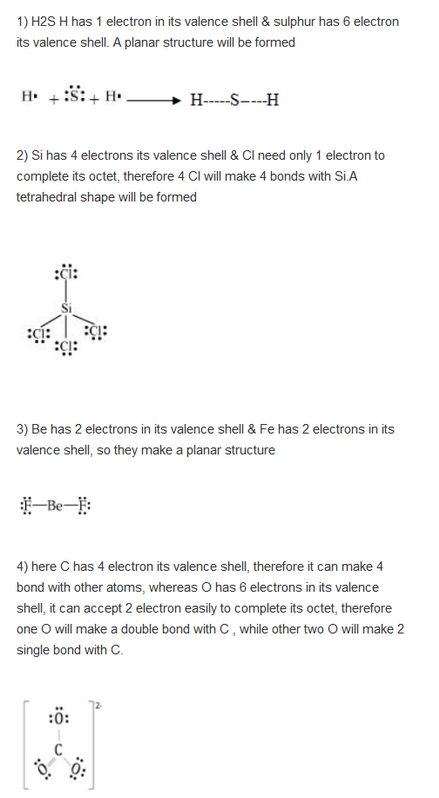5. Define octet rule. Write its significance and limitations.

Solution :

The octet rule or the electronic theory of chemical bonding was developed by Kossel and Lewis. According to this rule, atoms can combine either by transfer of valence electrons from one atom to another or by sharing their valence electrons in order to attain the nearest noble gas configuration by having an octet in their valence shell.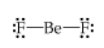The octet rule successfully explained the formation of chemical bonds depending upon the nature of the element.

Limitations of the octet theory:

The following are the limitations of the octet rule:

(a) The rule failed to predict the shape and relative stability of molecules.

(b) It is based upon the inert nature of noble gases. However, some noble gases like xenon and krypton form compounds such as XeF2, KrF2 etc.

(c) The octet rule cannot be applied to the elements in and beyond the third period of the periodic table. The elements present in these periods have more than eight valence electrons around the central atom. For example: PF5, SF6, etc.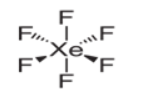(d) The octet rule is not satisfied for all atoms in a molecule having an odd number of electrons. For example, NO and NO2 do not satisfy the octet rule.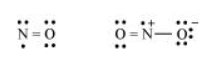(e) This rule cannot be applied to those compounds in which the number of electrons surrounding the central atom is less than eight. For example, LiCl, BeH2, AlCl3 etc. do not obey the octet rule.

6. Write the favourable factors for the formation of ionic bond.

Solution :

An ionic bond is formed by the transfer of one or more electrons from one atom to another. Hence, the formation of ionic bonds depends upon the ease with which neutral atoms can lose or gain electrons. Bond formation also depends upon the lattice energy of the compound formed.

Hence, favourable factors for ionic bond formation are as follows:

(i) Low ionization enthalpy of metal atom.

(ii) High electron gain enthalpy (Δeg H) of a non-metal atom.

(iii) High lattice energy of the compound formed.

7. Discuss the shape of the following molecules using the VSEPR model:

BeCl2, BCl3, SiCl4, AsF5, H2S, PH3

Solution :

BeCl2: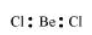The central atom has no lone pair and there are two bond pairs. i.e., BeCl2 is of the type AB2. Hence, it has a linear shape.

BCl3: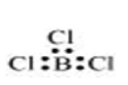The central atom has no lone pair and there are three bond pairs. Hence, it is of the type AB3. Hence, it is trigonal planar.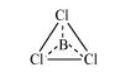SiCl4: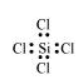The central atom has no lone pair and there are four bond pairs. Hence, the shape of SiCl4 is tetrahedral being the AB4 type molecule.

AsF5: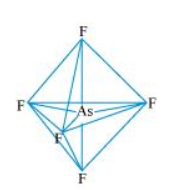The central atom has no lone pair and there are five bond pairs. Hence, AsF5 is of the type AB5. Therefore, the shape is trigonal bipyramidal.

H2S: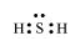The central atom has one lone pair and there are two bond pairs. Hence, H2S is of the type AB2E. The shape is Bent.

PH3: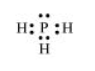The central atom has one lone pair and there are three bond pairs. Hence, PH3 is of the AB3E type. Therefore, the shape is trigonal pyramidal.

8. Although geometries of NH3 and H2O molecules are distorted tetrahedral, bond angle in water is less than that of ammonia. Discuss.

Solution :

The molecular geometry of NH3 and H2O can be shown as: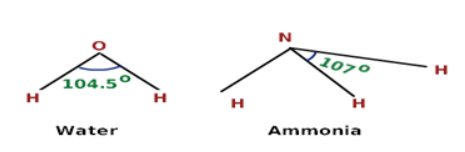The central atom (N) in NH3 has one lone pair and there are three bond pairs. In H2O, there are two lone pairs and two bond pairs.

The two lone pairs present in the oxygen atom of H2O molecule repels the two bond pairs. This repulsion is stronger than the repulsion between the lone pair and the three bond pairs on the nitrogen atom.

Since the repulsions on the bond pairs in H2O molecule are greater than that in NH3, the bond angle in water is less than that of ammonia.

9. How do you express the bond strength in terms of bond order?

Solution :

Bond strength represents the extent of bonding between two atoms forming a molecule. The larger the bond energy, the stronger is the bond and the greater is the bond order.

10. Define the bond length.

Solution :

Bond length is defined as the equilibrium distance between the nuclei of two bonded atoms in a molecule.

Bond lengths are expressed in terms of Angstrom (10–10 m) or picometer

(10–12 m) and are measured by spectroscopic X-ray diffractions and electron-diffraction techniques.

In an ionic compound, the bond length is the sum of the ionic radii of the constituting atoms (d = r+ + r). In a covalent compound, it is the sum of their covalent radii (d = rA + rB).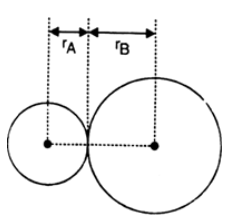11. Explain the important aspects of resonance with reference to the CO32– ion.

Solution :

According to experimental findings, all carbon to oxygen bonds in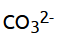are equivalent. Hence, it is inadequate to represention by a single Lewis structure having two single bonds and one double bond.

Therefore, carbonate ion is described as a resonance hybrid of the following structures: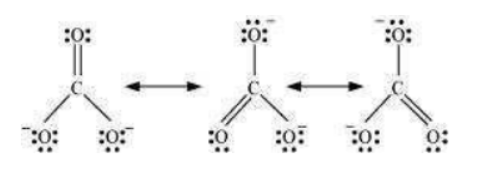12. H3PO3 can be represented by structures 1 and 2 shown below. Can these two structures be taken as the canonical forms of the resonance hybrid representing H3PO3? If not, give reasons for the same.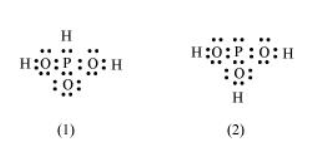Solution :

The given structures cannot be taken as the canonical forms of the resonance hybrid of H3PO3 because the positions of the atoms have changed.

13. Write the resonance structures for SO3, NO2 and NO3.

Solution :

The resonance structures are:

(a) SO3: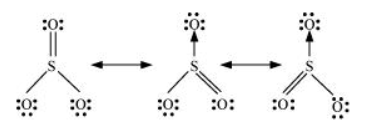(b)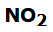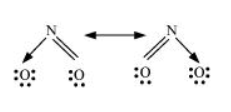(c)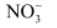: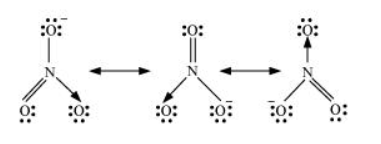14. Use Lewis symbols to show electron transfer between the following atoms to form cations and anions: (a) K and S (b) Ca and O (c) Al and N.

Solution :

(a) K and S:

The electronic configurations of K and S are as follows:

K: 2, 8, 8, 1

S: 2, 8, 6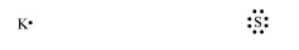Sulphur (S) requires 2 more electrons to complete its octet. Potassium (K) requires one electron more than the nearest noble gas i.e., Argon. Hence, the electron transfer can be shown as: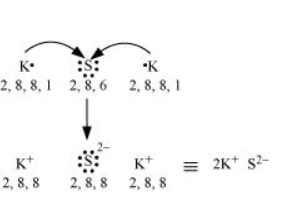(b) Ca and O:

The electronic configurations of Ca and O are as follows:

Ca: 2, 8, 8, 2

O: 2, 6

Oxygen requires two electrons more to complete its octet, whereas calcium has two electrons more than the nearest noble gas i.e., Argon. Hence, the electron transfer takes place as: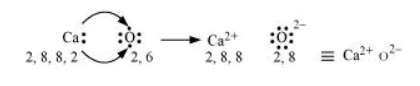(c) Al and N:

The electronic configurations of Al and N are as follows:

Al: 2, 8, 3

N: 2, 5

Nitrogen is three electrons short of the nearest noble gas (Neon), whereas aluminium has three electrons more than Neon. Hence, the electron transference can be shown as: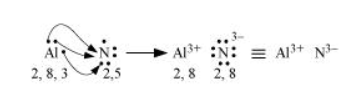15. Although both CO2 and H2O are triatomic molecules, the shape of H2O molecule is bent while that of CO2 is linear. Explain this on the basis of dipole moment.

Solution :

According to experimental results, the dipole moment of carbon dioxide is zero. This is possible only if the molecule is linear so that the dipole moments of C–O bonds are equal and opposite to nullify each other.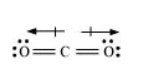Resultant μ = 0 D

H2O, on the other hand, has a dipole moment value of 1.84 D (though it is a triatomic molecule as CO2). The value of the dipole moment suggests that the structure of H2O molecule is bent where the dipole moment of O–H bonds are unequal.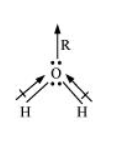16. Write the significance / applications of dipole moment.

Solution :

In heteronuclear molecules, polarization arises due to a difference in the electronegativities of the constituents of atoms. As a result, one end of the molecule acquires a positive charge while the other end becomes negative. Hence, a molecule is said to possess a dipole.

The product of the magnitude of the charge and the distance between the centres of positive-negative charges is called the dipole moment (μ) of the molecule. It is a vector quantity and is represented by an arrow with its tail at the positive centre and head pointing towards a negative centre.

Dipole moment (μ) = charge (Q) × distance of separation (r)

The SI unit of a dipole moment is ‘esu’.

1 esu = 3.335 × 10–30 Cm

Dipole moment is the measure of the polarity of a bond. It is used to differentiate between polar and non-polar bonds since all non-polar molecules (e.g. H2, O2) have zero dipole moments. It is also helpful in calculating the percentage ionic character of a molecule.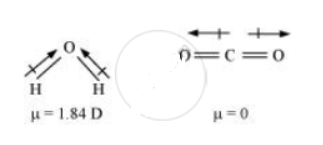17. Define electronegativity. How does it differ from electron gain enthalpy?

Solution :

Electronegativity is the ability of an atom in a chemical compound to attract a bond pair of electrons towards itself.

Electronegativity of any given element is not constant. It varies according to the element to which it is bound. It is not a measurable quantity. It is only a relative number.

On the other hand, electron gain enthalpy is the enthalpy change that takes place when an electron is added to a neutral gaseous atom to form an anion. It can be negative or positive depending upon whether the electron is added or removed. An element has a constant value of the electron gain enthalpy that can be measured experimentally.

18. Explain with the help of suitable example polar covalent bond.

Solution :

When two dissimilar atoms having different electronegativities combine to form a covalent bond, the bond pair of electrons is not shared equally. The bond pair shifts towards the nucleus of the atom having greater electronegativity. As a result, electron distribution gets distorted and the electron cloud is displaced towards the electronegative atom.

As a result, the electronegative atom becomes slightly negatively charged while the other atom becomes slightly positively charged. Thus, opposite poles are developed in the molecule and this type of a bond is called a polar covalent bond.

HCl, for example, contains a polar covalent bond. Chlorine atom is more electronegative than hydrogen atom. Hence, the bond pair lies towards chlorine and therefore, it acquires a partial negative charge.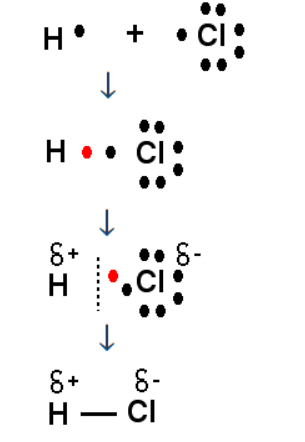19. Arrange the bonds in order of increasing ionic character in the molecules: LiF, K2O, N2, SO2 and ClF3.

Solution :

The ionic character in a molecule is dependent upon the electronegativity difference between the constituting atoms. The greater the difference, the greater will be the ionic character of the molecule.

On this basis, the order of increasing ionic character in the given molecules is

N2 < SO2 < ClF3 < K2O < LiF.

20. The skeletal structure of CH3COOH as shown below is correct, but some of the bonds are shown incorrectly. Write the correct Lewis structure for acetic acid.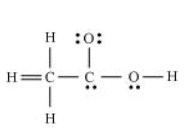Solution :

The correct Lewis structure for acetic acid is as follows: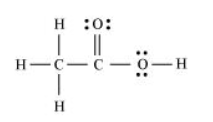21. Apart from tetrahedral geometry, another possible geometry for CH4 is square planar with the four H atoms at the corners of the square and the C atom at its centre. Explain why CH4 is not square planar?

Solution :

Electronic configuration of carbon atom:

6C: 1s2 2s2 2p2

In the excited state, the orbital picture of carbon can be represented as: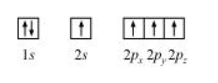Hence, carbon atom undergoes sp3 hybridization in CH4 molecule and takes a tetrahedral shape.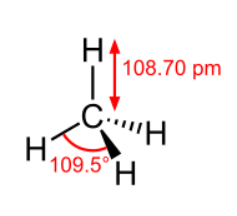For a square planar shape, the hybridization of the central atom has to be dsp2. However, an atom of carbon does not have d-orbitalsto undergo dsp2 hybridization. Hence, the structure of CH4 cannot be square planar.

Moreover, with a bond angle of 90° in square planar, the stability of CH4 will be very less because of the repulsion existing between the bond pairs. Hence, VSEPR theory also supports a tetrahedral structure for CH4.

22. Explain why BeH2 molecule has a zero dipole moment although the Be–H bonds are polar.

Solution :

The Lewis structure for BeH2 is as follows: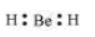There is no lone pair at the central atom (Be) and there are two bond pairs. Hence, BeH2 is of the type AB2. It has a linear structure.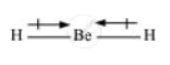Dipole moments of each H–Be bond are equal and are in opposite directions. Therefore, they nullify each other. Hence, BeH2 molecule has zero dipole moment.

23. Which out of NH3 and NF3 has higher dipole moment and why?

Solution :

In both molecules i.e., NH3 and NF3, the central atom (N) has a lone pair electron and there are three bond pairs. Hence, both molecules have a pyramidal shape. Since fluorine is more electronegative than hydrogen, it is expected that the net dipole moment of NF3 is greater than NH3. However, the net dipole moment of NH3 (1.46 D) is greater than that of NF3 (0.24 D).

This can be explained on the basis of the directions of the dipole moments of each individual bond in NF3 and NH3. These directions can be shown as: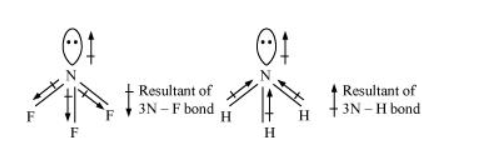Thus, the resultant moment of the N–H bonds add up to the bond moment of the lone pair (the two being in the same direction), whereas that of the three N – F bonds partly cancels the moment of the lone pair.

Hence, the net dipole moment of NF3 is less than that of NH3.

24. What is meant by hybridisation of atomic orbitals? Describe the shapes of sp, sp2, sp3 hybrid orbitals.

Solution :

Hybridization is defined as an intermixing of a set of atomic orbitals of slightly different energies, thereby forming a new set of orbitals having equivalent energies and shapes.

For example, one 2s-orbital hybridizes with two 2p-orbitals of carbon to form three new sp2 hybrid orbitals.

These hybrid orbitals have minimum repulsion between their electron pairs and thus, are more stable. Hybridization helps indicate the geometry of the molecule.

Shape of sp hybrid orbitals: sp hybrid orbitals have a linear shape.

They are formed by the intermixing of s and p orbitals as: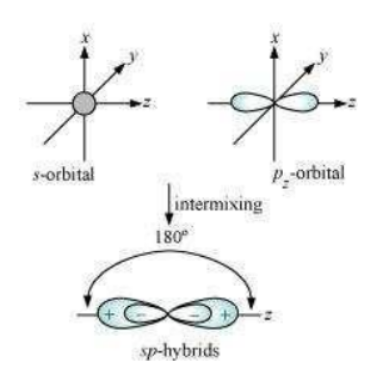Shape of sp2 hybrid orbitals:

sp2 hybrid orbitals are formed as a result of the intermixing of one s-orbital and two 2p-orbitals. The hybrid orbitals are oriented in a trigonal planar arrangement as: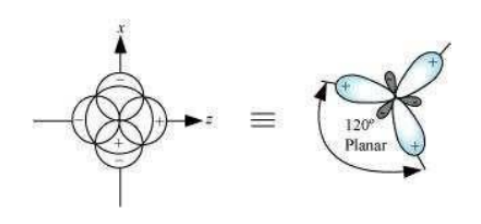Shape of sp3 hybrid orbitals:

Four sp3 hybrid orbitals are formed by intermixing one s-orbital with three p-orbitals.

The four sp3 hybrid orbitals are arranged in the form of a tetrahedron as: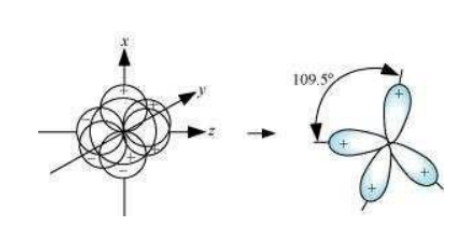25. Describe the change in hybridisation (if any) of the Al atom in the following reaction.

AlCl3 +  Cl- → AlCl-4

Solution :

The valence orbital picture of aluminium in the ground state can be represented as: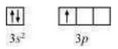The orbital picture of aluminium in the excited state can be represented as: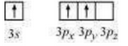Hence, it undergoes sp2 hybridization to give a trigonal planar arrangement (in AlCl3).

To form AlCl4, the empty 3pz orbital also gets involved and the hybridization changes from sp2 to sp3. As a result, the shape gets changed to tetrahedral.

26. Is there any change in the hybridisation of B and N atoms as a result of the following reaction?

BF3 + NH3 → F3B.NH3

Solution :

Boron atom in BF3 is sp2 hybridized. The orbital picture of boron in the excited state can be shown as: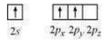Nitrogen atom in NH3 is sp3 hybridized. The orbital picture of nitrogen can be represented as: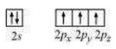After the reaction has occurred, an adduct F3B⋅NH3 is formed as hybridization of ‘B’ changes to sp3. However, the hybridization of ‘N’ remains intact.

27. Draw diagrams showing the formation of a double bond and a triple bond between carbon atoms in C2H4 and C2H2 molecules.

Solution :

C2H4 :

The electronic configuration of C-atom in the excited state is: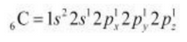In the formation of an ethane molecule (C2H4), one sp2 hybrid orbital of carbon overlaps a sp2 hybridized orbital of another carbon atom, thereby forming a C-C sigma bond.

The remaining two sp2 orbitals of each carbon atom form a sp2-s sigma bond with two hydrogen atoms. The unhybridized orbital of one carbon atom undergoes sidewise overlap with the orbital of a similar kind present on another carbon atom to form a weak π-bond.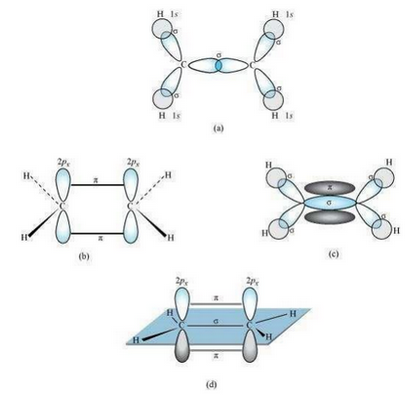C2H2 :

In the formation of C2H2 molecule, each C–atom is sp hybridized with two 2p-orbitals in an unhybridized state.

One sp orbital of each carbon atom overlaps with the other along the internuclear axis forming a C–C sigma bond. The second sp orbital of each C–atom overlaps a half-filled 1s-orbital to form a σ bond.

The two unhybridized 2p-orbitals of the first carbon undergo sidewise overlap with the 2p orbital of another carbon atom, thereby forming two pi (π) bonds between carbon atoms. Hence, the triple bond between two carbon atoms is made up of one sigma and two π-bonds.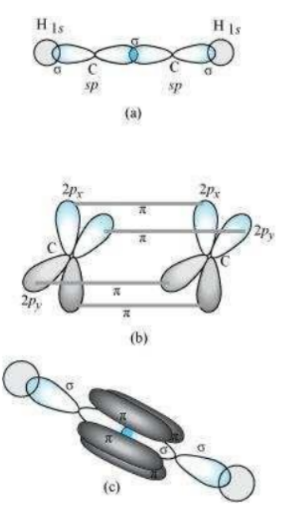28. What is the total number of sigma and pi bonds in the following molecules?

(a) C2H2 (b) C2H4

Solution :

A single bond is a result of the axial overlap of bonding orbitals. Hence, it contributes a sigma bond. A multiple bond (double or triple bond) is always formed as a result of the sidewise overlap of orbitals. A pi-bond is always present in it. A triple bond is a combination of two pi-bonds and one sigma bond.

Structure of C2H2 can be represented as: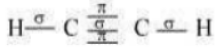Hence, there are three sigma and two pi-bonds in C2H2.

The structure of C2H4 can be represented as: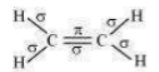Hence, there are five sigma bonds and one pi-bond in C2H4.

29. Considering x-axis as the internuclear axis which out of the following will not form a sigma bond and why? (a) 1s and 1s (b) 1s and 2px (c) 2py and 2py (d) 1s and 2s.

Solution :

2py and 2py orbitals will not a form a sigma bond. Taking x-axis as the internuclear axis, 2py and 2py orbitals will undergo lateral overlapping, thereby forming a pi (π) bond.

30. Which hybrid orbitals are used by carbon atoms in the following molecules?

CH3–CH3; (b) CH3–CH=CH2; (c) CH3-CH2-OH; (d) CH3-CHO (e) CH3COOH

Solution :

(a)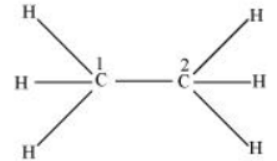Both C1 and C2 are sp3 hybridized.

(b)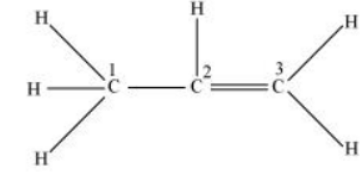C1 is sp3 hybridized, while C2 and C3 are sp2 hybridized.

(c)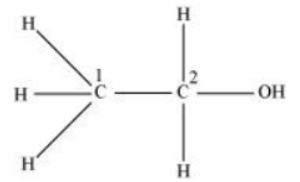Both C1 and C2 are sp3 hybridized.

(d)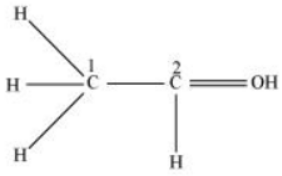C1 is sp3 hybridized and C2 is sp2 hybridized.

(e)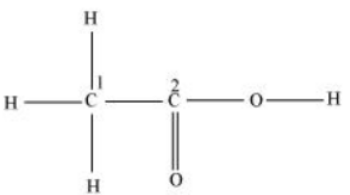C1 is sp3 hybridized and C2 is sp2 hybridized.

31. What do you understand by bond pairs and lone pairs of electrons? Illustrate by giving one example of each type.

Solution :

When two atoms combine by sharing their one or more valence electrons, a covalent bond is formed between them.

The shared pairs of electrons present between the bonded atoms are called bond pairs. All valence electrons may not participate in bonding. The electron pairs that do not participate in bonding are called lone pairs of electrons.

For example, in C2H6 (ethane), there are seven bond pairs but no lone pair present.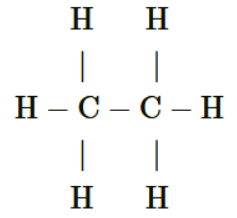In H2O, there are two bond pairs and two lone pairs on the central atom (oxygen).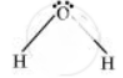32. Distinguish between a sigma and a pi bond.

Solution :

The following are the differences between sigma and pi-bonds:

 Sigma (σ) Bond Pi (π) Bond (a) It is formed by the end to end overlap of orbitals. It is formed by the lateral overlap of orbitals. (b) The orbitals involved in the overlapping are s–s, s–p, or p–p. These bonds are formed by the overlap of p–p orbitals only. (c) It is a strong bond. It is weak bond. (d) The electron cloud is symmetrical about the line joining the two nuclei. The electron cloud is not symmetrical. (e) It consists of one electron cloud, which is symmetrical about the internuclear axis. There are two electron clouds lying above and below the plane of the atomic nuclei. (f) Free rotation about σ bonds is possible. Rotation is restricted in case of pi-bonds.

33. Explain the formation of H2 molecule on the basis of valence bond theory.

Solution :

Let us assume that two hydrogen atoms (A and B) with nuclei (NA and NB) and electrons (eA and eB) are taken to undergo a reaction to form a hydrogen molecule.

When A and B are at a large distance, there is no interaction between them. As they begin to approach each other, the attractive and repulsive forces start operating.

Attractive force arises between:

(a) Nucleus of one atom and its own electron i.e., NA – eA and NB – eB.

(b) Nucleus of one atom and electron of another atom i.e., NA – eB and NB – eA.

Repulsive force arises between:

(a) Electrons of two atoms i.e., eA – eB.

(b) Nuclei of two atoms i.e., NA – NB.

The force of attraction brings the two atoms together, whereas the force of repulsion tends to push them apart.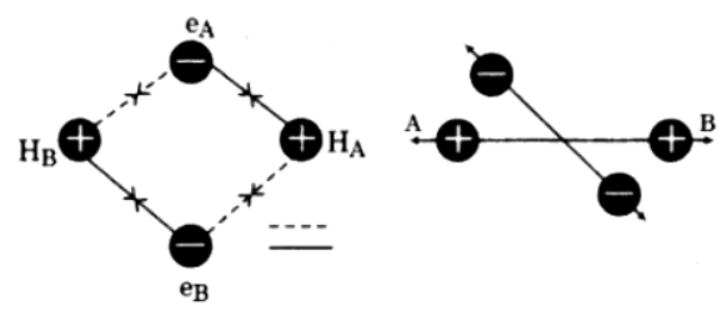The magnitude of the attractive forces is more than that of the repulsive forces. Hence, the two atoms approach each other. As a result, the potential energy decreases. Finally, a state is reached when the attractive forces balance the repulsive forces and the system acquires minimum energy. This leads to the formation of a dihydrogen molecule.

34. Write the important conditions required for the linear combination of atomic orbitals to form molecular orbitals.

Solution :

The given conditions should be satisfied by atomic orbitals to form molecular orbitals:

(a) The combining atomic orbitals must have the same or nearly the same energy. This means that in a homonuclear molecule, the 1s-atomic orbital of an atom can combine with the 1s-atomic orbital of another atom, and not with the 2s-orbital.

(b) The combining atomic orbitals must have proper orientations to ensure that the overlap is maximum.

(c) The extent of overlapping should be large.

35. Use molecular orbital theory to explain why the Be2 molecule does not exist.

Solution :

The electronic configuration of Beryllium is 1s2  2s2

The molecular orbital electronic configuration for Be2 molecule can be written as: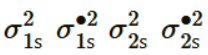Hence, the bond order for Be2 is 1/2(Nb - Na)

Where,

Nb = Number of electrons in bonding orbitals

Na = Number of electrons in anti-bonding orbitals

∴ Bond order of Be2 1/2(4 - 4) = 0

A negative or zero bond order means that the molecule is unstable. Hence, Be2 molecule does not exist.

36. Compare the relative stability of the following species and indicate their magnetic properties;

O2,O2+,O2- (superoxide), O22- (peroxide)

Solution :

There are 16 electrons in a molecule of dioxygen, 8 from each oxygen atom. The electronic configuration of oxygen molecule can be written as: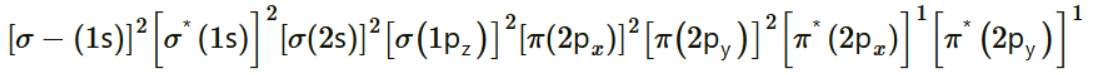Since the 1s orbital of each oxygen atom is not involved in boding, the number of bonding electrons = 8 = Nb and the number of anti-bonding orbitals = 4 = Na.

Bond order 1/2(Nb - Na)

1/2(8 - 4) = 2

Similarly, the electronic configuration of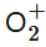can be written as: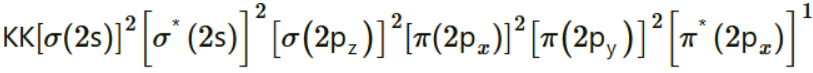Nb = 8

Na = 3

Bond order of= 1/2 (8 - 3)

= 2.5

Electronic configuration of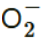ion will be: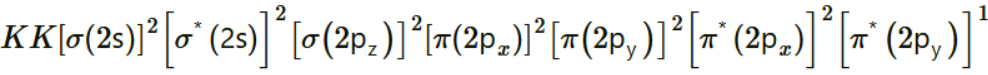Nb = 8

Na = 5

Bond order of= 1/2(8 -5)

= 1.5

Electronic configuration of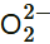ion will be: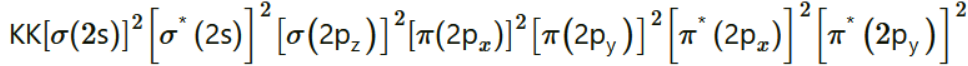Nb = 8

Na = 6

Bond order of= 1/2(8 - 6)

= 1

Bond dissociation energy is directly proportional to bond order. Thus, the higher the bond order, the greater will be the stability. On this basis, the order of stability is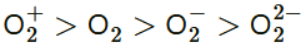.

37. Write the significance of a plus and a minus sign shown in representing the orbitals.

Solution :

Molecular orbitals are represented by wave functions. A plus sign in an orbital indicates a positive wave function while a minus sign in an orbital represents a negative wave function.

38. Describe the hybridisation in case of PCl5. Why are the axial bonds longer as compared to equatorial bonds?

Solution :

The ground state and excited state outer electronic configurations of phosphorus (Z = 15) are: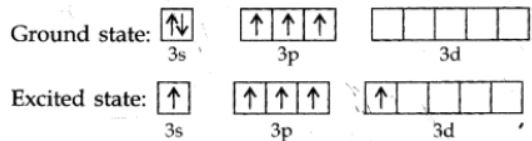Phosphorus atom is sp3d hybridized in the excited state. These orbitals are filled by the electron pairs donated by five Cl atoms as:

PCl5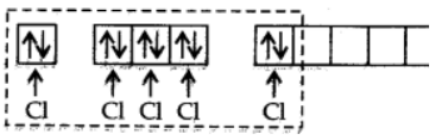The five sp3d hybrid orbitals are directed towards the five corners of the trigonal bipyramidals. Hence, the geometry of PCl5 can be represented as: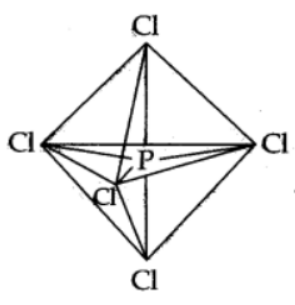There are five P–Cl sigma bonds in PCl5. Three P–Cl bonds lie in one plane and make an angle of 120° with each other. These bonds are called equatorial bonds.

The remaining two P–Cl bonds lie above and below the equatorial plane and make an angle of 90° with the plane. These bonds are called axial bonds.

As the axial bond pairs suffer more repulsion from the equatorial bond pairs, axial bonds are slightly longer than equatorial bonds.

39. Define hydrogen bond. Is it weaker or stronger than the van der Waals forces?

Solution :

A hydrogen bond is defined as an attractive force acting between the hydrogen attached to an electronegative atom of one molecule and an electronegative atom of a different molecule (may be of the same kind).

Due to a difference between electronegativities, the bond pair between hydrogen and the electronegative atom gets drifted far away from the hydrogen atom. As a result, a hydrogen atom becomes electropositive with respect to the other atom and acquires a positive charge.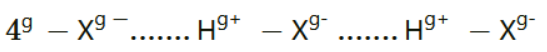The magnitude of H-bonding is maximum in the solid state and minimum in the gaseous state.

There are two types of H-bonds:

(i) Intermolecular H-bond e.g., HF, H2O etc.

(ii) Intramolecular H-bond e.g., o-nitrophenol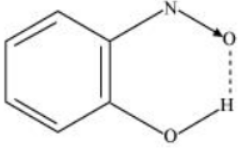Hydrogen bonds are stronger than Van der Walls forces since hydrogen bonds are regarded as an extreme form of dipole-dipole interaction.

40. What is meant by the term bond order? Calculate the bond order of: N2, O2,O2+ and O2-.

Solution :

Bond order is defined as one half of the difference between the number of electrons present in the bonding and anti-bonding orbitals of a molecule.

If Na is equal to the number of electrons in an anti-bonding orbital, then Nb is equal to the number of electrons in a bonding orbital.

Bond order = [no. of electrons in bonding MO - no. of electrons in antibonding MO]/2

If Nb > Na, then the molecule is said be stable. However, if Nb ≤ Na, then the molecule is considered to be unstable.

Bond order of N2 can be calculated from its electronic configuration as: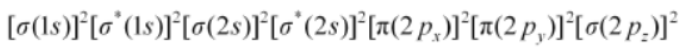Number of bonding electrons, Nb = 10

Number of anti-bonding electrons, Na = 4

Bond order of nitrogen molecule 1/2(10 - 4) = 3

There are 16 electrons in a dioxygen molecule, 8 from each oxygen atom. The electronic configuration of oxygen molecule can be written as: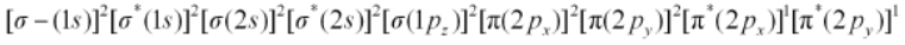Since the 1s orbital of each oxygen atom is not involved in boding, the number of bonding electrons = 8 = Nb and the number of anti-bonding electrons = 4 = Na.

Bond order 1/2(8-4)

= 2

Hence, the bond order of oxygen molecule is 2.

Similarly, the electronic configuration ofcan be written as:Nb = 8

Na = 3

Bond order of 1/2(8 - 5)

= 2.5

Thus, the bond order ofis 2.5.

The electronic configuration ofion will be:Nb = 8

Na = 5

Bond order of 1/2(8 - 5)

= 1.5

Thus, the bond order ofion is 1.5.